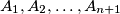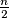### IMO Shortlist 1988 problem 5

Kvaliteta:
Avg: 0,0
Težina:
Avg: 0,0
Let$n$ be an even positive integer. Let$A_1, A_2, \ldots, A_{n + 1}$ be sets having$n$ elements each such that any two of them have exactly one element in common while every element of their union belongs to at least two of the given sets. For which$n$ can one assign to every element of the union one of the numbers 0 and 1 in such a manner that each of the sets has exactly$\frac {n}{2}$ zeros?
Izvor: Međunarodna matematička olimpijada, shortlist 1988